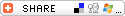## PHP - Improve object to scalar conversion

Projects -
(4 votes, average 4.25 out of 5)The __toString() magic method allows to convert an object to a string. This mechanism can be extended to allow converting to other scalar types. New rules can also allow converting the value, even when the specific method is not implemented.

## New magic methods

The first part is easy to understand : we add three new magic methods : __toBool(), __toInt(), and __toFloat().

## New conversion rules

When converting an objet to scalar, the conversion logic will be :

• Does a magic method to convert to the destination type exist ? If yes, convert using this method.
• Use a fallback mechanism to search alternate magic methods. When one is found, use it and then convert this interim result to the destination type. If this conversion fails, search the newt one.

Here are the methods that will be searched depending on the target type :

Target Try these methods in turn...
Bool Bool Int Float String
Int Int Float String Bool
Float Float Int String Bool
String String Int Float Bool

In case of a two-step conversion, scalar conversion rules apply.

Examples

 ```class Foo { public function __toInt() {     return 10; } } //----------------- \$obj=new Foo(); var_dump(\$obj + 1);    // -> int(10) var_dump(\$obj.'a');    // -> string(3) "10a" echo (\$obj ? 'true' : 'false')    // -> true```

Conversion can fail, even when a magic method is found :

 ```class Foo { public function __toString() { return '\$ 25'; } } //----------------- \$obj=new Foo(); var_dump(\$obj + 1);    // Failure "\$ 25" cannot be converted to int```

When a method fails, we try the next one, if any :

 ```class Foo { public function __toString() { return '\$ 25'; } public function __toBool() { return true; } } //----------------- \$obj=new Foo(); var_dump(\$obj + 1);    // -> int(2) (__toBool() was used after string -> int conversion failed)```